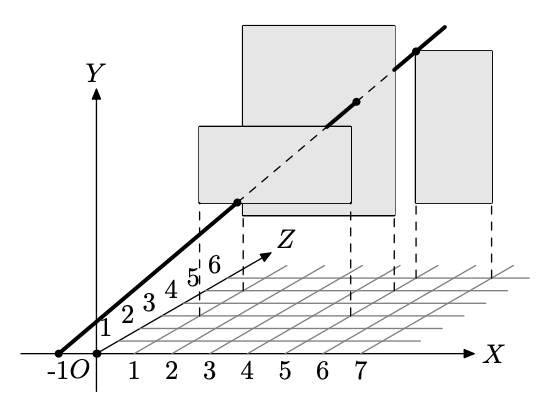시간 제한 메모리 제한 제출 정답 맞은 사람 정답 비율
2 초 128 MB 0 0 0 0.000%

## 문제

Consider a 3D scene with $OXY\!Z$ coordinate system. Axis $OX$ points to the right, axis $OY$ points up, and axis $OZ$ points away from you. There is a number of rectangular windows on the scene. The plane of each window is parallel to $OXY$, its sides are parallel to $OX$ and $OY$. All windows are situated at different depths on the scene (different coordinates $z > 0$).A gunman with a rifle moves along $OX$ axis ($y = 0$ and $z = 0$). He can shoot a bullet in a straight line. His goal is to shoot a single bullet through all the windows. Just touching a window edge is enough.

## 입력

The first line of the input file contains a single integer number $n$ ($2 \le n \le 100$) --- the number of windows on the scene. The following $n$ lines describe the windows. Each line contains five integer numbers $x_{1i}$, $y_{1i}$, $x_{2i}$, $y_{2i}$, $z_i$ ($0 < x_{1i}$, $y_{1i}$, $x_{2i}$, $y_{2i}$, $z_i < 1000$). Here $(x_{1i}, y_{1i}, z_i)$ are coordinates of the bottom left corner of the window, and $(x_{2i}, y_{2i}, z_i)$ are coordinates of the top right corner of the window ($x_{1i} < x_{2i}$, $y_{1i} < y_{2i}$). Windows are ordered by $z$ coordinate ($z_i > z_{i-1}$ for $2 \le i \le n$).

## 출력

Output a single word "UNSOLVABLE" if the gunman cannot reach the goal of shooting a bullet through all the windows.

Otherwise, on the first line output a word "SOLUTION". On the next line output $x$ coordinate of the point from which the gunman must fire a bullet. On the following $n$ lines output $x, y, z$ coordinates of the points where the bullet goes through the consecutive windows. All coordinates in the output file must be printed with six digits after decimal point.

## 예제 입력 1

3
1 3 5 5 3
1 2 5 7 5
5 2 7 6 6


## 예제 출력 1

SOLUTION
-1.000000
2.000000 3.000000 3.000000
4.000000 5.000000 5.000000
5.000000 6.000000 6.000000


## 예제 입력 2

3
2 1 5 4 1
3 5 6 8 2
4 3 8 6 4


## 예제 출력 2

UNSOLVABLE